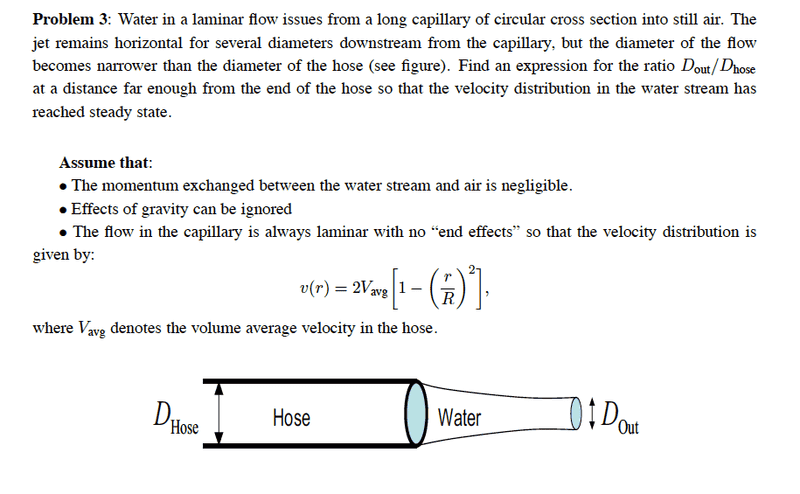# Fluid mechanics problem involving a hose and water coming out.

guitar24

## Homework Statement## Homework Equations

I am having some trouble starting this problem. At first look I would say that a conservation of mass will give V1*A1=V2*A2 for steady state incompressible fluid. Then I would solve for A2 and relate the two diameters but I don't have the velocity distribution of the water stream. Any hints??

## Answers and Replies

Homework Helper
Gold Member
You're spot on to include conservation of volume, but V1*A1 = V2*A2 can't be right since the flow of water thru A1 varies with r. So think of the total volume of water flowing in 1 sec. thru A1 and equate that to the total volume of water flowing in 1 sec. thru A2.

guitar24
Thank you for your reply! could you elaborate a bit more on how I would do that?
The total volumetric flow rate in the tube is Vavg*Area of the tube. Multiply by one and you get the volume of water entering the cross section in one second. Then i would just divide that by the area of the water stream to get the volume of water flowing in one sec through the area of the water stream. Then just rearrange the equation to get Dout/Dhose. Is that correct?

Thank you!

Homework Helper
Gold Member
Thank you for your reply! could you elaborate a bit more on how I would do that?
The total volumetric flow rate in the tube is Vavg*Area of the tube. Multiply by one and you get the volume of water entering the cross section in one second. Then i would just divide that by the area of the water stream to get the volume of water flowing in one sec through the area of the water stream. Then just rearrange the equation to get Dout/Dhose. Is that correct?

Thank you!

I computed the average flow per unit time and found it to be not Vavg but (1/R)∫v(r)dr with limits 0 and R, = (4/3)Vavg which is somewhat weird.

Anyway, whatever average V is, you are right in using mass conservation flow to get the answer. But you still need to get v, the (uniform) velocity of the stream exiting at Dout. For that, go to Bernoulli.

LawrenceC
Given the assumption that there is no interaction with the surrounding air and thus no momentum loss, that suggests momentum is constant. Write integrals representing the momentum at the nozzle using the given velocity profile and one for the region downstream (utilizing conservation of mass flow) where the velocity is uniform. Equate them and solve for diameter ratios.